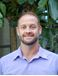Sale ends in
Teacher: Josh
Customers Who Have Viewed This Course: 3900
\$249.99
\$104.00

#### 0 Course Introduction 01:00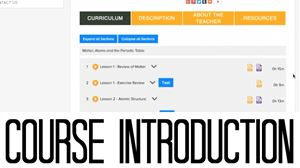Course Includes

• Over 10 hours of video content

• 25 video lessons

• 25 worksheet review videos

• 25 practice worksheets

#### 1 Lesson 1 - Review of Matter 15:49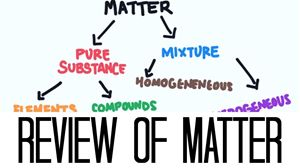This is the first lesson in grade 11 chemistry. This lesson is called Review of Matter.

I split this lesson down into 3 parts.

The first part is called Classifying Matter, where we will learn the difference between a pure substance and a mixture and then learn about different types of mixtures.

the second part is called Properties of Matter, where we will learn about different types of properties and different ways that matter can change.

and the third part is called Density. We will focus on one important property of matter called density that compares the mass of a substance to its volume.

#### 2 Lesson 1 - Exercise Review 10:22

This video will review the worksheet for Lesson 1 - Review of Matter.

#### 3 Lesson 2 - Atomic Structure 13:45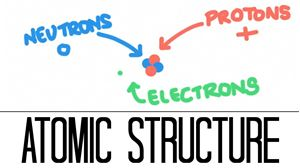This is a two part lesson called Atomic Structure.

The first part is called “The Atom”, where we will learn about the subatomic particles protons, neutrons and electrons.

The second part is called “Isotopes”, where we will learn what isotopes are and how to calculate the atomic weight of an element.

So good luck and have fun learning about the atom!

#### 4 Lesson 2 - Exercise Review 08:38

This video will review the worksheet for Lesson 2.

#### 5 Lesson 3 - Ions 09:08This is a one part lesson called Ions.

We will learn what an ion is, how atoms become ions and then we will learn about different types of ions.

So good luck and have fun learning about ions!

#### 6 Lesson 3 - Exercise Review 07:10

This video will review the worksheet for Lesson 3.

#### 7 Lesson 4 - The Periodic Table 15:23This is a three part lesson called, The Periodic Table

The first part is called “Organization of the Elements”, where we will learn about where the periodic table came from and how the periodic table organizes elements by common properties.

The second part is called “Chemical Families”, where we will learn about elements that share similar physical and chemical properties.

The third part is called “The Alkali Metals”, where we will look a little closer at one of the chemical families on the periodic table and get see a real experiment and see how the alkali metals react with water.

So good luck and have fun learning about the periodic table!

#### 8 Lesson 4 - Exercise Review 08:11

This video will review the worksheet for Lesson 4.

#### 9 Lesson 5 - Periodic Trends 13:57This is a three part lesson called, Periodic Trends.

The first part is called “Atomic Radius” where we will learn how the size of an atom is measured and then learn how we can predict the relative size of an atom.

The second part is called “Electronegativity” where we will learn what electronegativity is and how we can predict the relative electronegativity of an element.

The third part is called “Ionization Energy” where we will learn what ionization is and how we can predict the relative ionization energy of an element.

So good luck and have fun learning about periodic trends!

#### 10 Lesson 5 - Exercise Review 10:29

This video will review the worksheet for Lesson 5.

#### 11 Lesson 6 - Ionic Bonding and Compounds 15:54This is a three part lesson called Ionic Bonding and Compounds.

The first part is called Properties of Ionic Compounds. We will explore some of the interesting characteristics of ionic compounds.

The second part is called Ionic Bonds, In this part we will learn how ionic bonds form.

The third part is called Crystal Structures, where we will take a closer look at how atoms arrange themselves inside of an ionic compound.

So, good luck and have fun learning about Ionic bonding and compounds!

#### 12 Lesson 6 - Exercise Review 07:57

This video will review the worksheet for Lesson 6.

#### 13 Lesson 7 - Ionic Compound Nomenclature 20:19This is a one part lesson called Ionic Compound Nomenclature where we will learn how to name three different types of ionic compounds.

So good luck and have fun learning about ionic compound nomenclature!

#### 14 Lesson 7 - Exercise Review 10:28

In this video we review the worksheet for lesson 7.

#### 15 Lesson 8 - Covalent Bonding and Compounds 18:22This is a three part lesson called Covalent bonding and compounds.

The first part is called properties of covalent compounds. We will explore some of the characteristics of covalent compounds and see how they are different from ionic compounds.

The second part is called Covalent bonds and structural formula. Here we will learn how to draw a structural formul and determine the molecular formula of a covalent compound.

The third part is called Covalent Compound Nomenclature. In this part we will learn how to name covalent compounds.

So good luck and have fun learning about covalent bonding and compounds!

#### 16 Lesson 8 - Exercise Review 10:01

In this video we review the worksheet for Lesson 8.

#### 17 Lesson 9 - Special Covalent Bonding 06:30This is a two part lesson called Special Covalent Bonding where we will learn about some of the unique ways that atoms and covalently bond to form compounds.

In the first part we will look at single, double and triple bonds

In the second part we will look at coordinate covalent bonds

So good luck and have fun learning about special covalent bonds!

#### 18 Lesson 9 - Exercise Review 07:32

In this video we review the worksheet for Lesson 9.

#### 19 Lesson 10 - Intermolecular Forces 08:54This is a one part lesson called Intermolecular Forces where we will learn how covalent compounds can be polar or nonpolar and then learn about the forces of attraction between chemical compounds.

So good luck and have fun learning about intermolecular forces!

#### 20 Lesson 10 - Exercise Review 13:09

In this video we review the worksheet for Lesson 10.

#### 21 Lesson 11 - Balancing Chemical Equations 09:16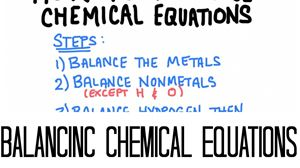This is a one part lesson called Balancing Chemical Equations, where will learn how to balance chemical equations according to the law of conservation of mass.

So, good luck and have fun learning how to balance chemical equations!

#### 22 Lesson 11 - Exercise Review 12:07

In this video we review the worksheet for lesson 11.

#### 23 Lesson 12 - Types of Chemical Reactions (Part 1) 21:46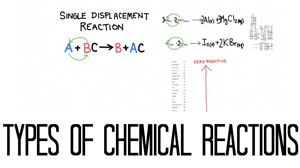This is a five part lesson called types of chemical reactions.

Each part will consist of a different type of chemical reaction. In each part we will learn about that type of reaction and how to predict the products of a chemical reaction.

So, good luck and have fun learning about types of chemical reactions!

#### 24 Lesson 12 - Types of Chemical Reactions (Part 2) 16:01This is a five part lesson called types of chemical reactions.

Each part will consist of a different type of chemical reaction. In each part we will learn about that type of reaction and how to predict the products of a chemical reaction.

So, good luck and have fun learning about types of chemical reactions!

#### 25 Lesson 12 - Exercise Review 15:30

In this video we review the worksheet for Lesson 12.

#### 26 Lesson 13 - Moles and Molar Mass of Compounds 15:33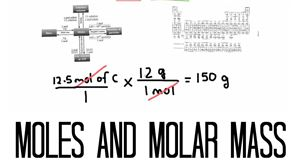This is a two part lesson called Moles and Molar Mass of Compounds.

The first part is called Moles, and in this part we will learn about the standard unit of measure of chemistry called the Mole.

The second part is called Converting the Mole. In this part we will learn how to convert between the mole and other common units of measure like grams and litres.

So, good luck and have fun learning about Moles and Molar Mass of Compounds!

#### 27 Lesson 13 - Exercise Review 12:51

In this video we review the worksheet for Lesson 13.

#### 28 Lesson 14 - Percent Composition, Empirical and Molecular Formula 23:54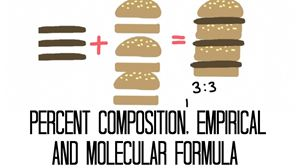This is a three part lesson called Percent Composition, Empirical and Molecular formula.

The first part is called percent composition. In this part we will learn how to determine the percentage of each element in a compound.

The second part is called Empirical Formula. We will learn how to determine the empirical formula of a chemical compound.

The Third part is called molecular formula. We will learn how to determine the molecular formula of a compound based on the empirical formula and the molar mass of the compound.

So good luck and have fun learning about percent composition, empirical and molecular formula!

#### 29 Lesson 14 - Exercise Review 12:19

In this video we review the worksheet for Lesson 14.

#### 30 Lesson 15 - Stoichiometry 29:58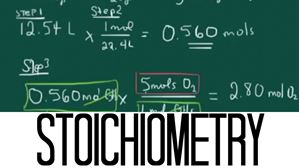This is a three part lesson called stoichiometry, where we will learn how stoichiometry is used to predict amounts of products formed or reactants used up during chemical reactions.

So, good luck and have fun learning about stoichiometry!

#### 31 Lesson 15 - Exercise Review 10:43

In this video we review the worksheet for Lesson 15.

#### 32 Lesson 16 - Limiting Reactants and Percent Yield 14:27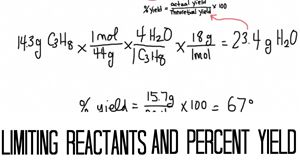This is a two part lesson called Limiting Reactant and Percent Yield.

The first part is called limiting reactants. In this part we will learn how to determine the limiting reactant in a chemical reaction and then determine the amount of product that could be created.

So good luck and have fun learning about limiting reactants and percent yield!

#### 33 Lesson 16 - Exercise Review 09:24

In this video we review the worksheet for Lesson 16.

#### 34 Lesson 17 - Kinetic Theory and Temperature 29:58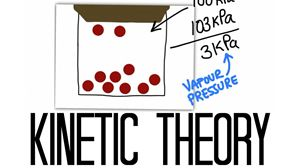This is a four part lesson called Kinetic Theory, Temperature and Pressure.

The first part is called Kinetic Theory, where we will learn about how molecules behave in a solid liquid and gas.

The second part is called Pressure, we will learn what pressure is and how to convert between common units of pressure.

The third part is called “Changing State” we will learn how a substance changes state between solid, liquid and gas.

The fourth part is called phase diagrams. Here we will learn how temperature and pressure are involved in the phase changing process.

So good luck and have fun learning about Kinetic theory, temperature and pressure!

#### 35 Lesson 17 - Exercise Review 12:59

In this video we review the worksheet for Lesson 17.

#### 36 Lesson 18 - The Gas Laws 13:15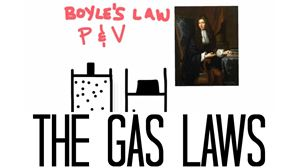This is a one part lesson called the gas laws.

In this lesson we will learn about 5 different gas laws. We will learn equations that describe these laws and learn how to use these equations.

So good luck and have fun learning about the gas laws!

#### 37 Lesson 18 - Exercise Review 11:18

In this video we review the worksheet for Lesson 18.

#### 38 Lesson 19 - The Ideal Gas Law 07:10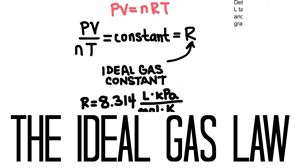This is a one part lesson called the Ideal Gas Law, where we will learn what an ideal gas is and the learn how to use the ideal gas law equation.

So good luck and have fun learning about the ideal gas law!

#### 39 Lesson 19 - Exercise Review 11:02

In this video we review the worksheet for Lesson 19.

#### 40 Lesson 20 - Partial Pressure and Effusion 15:28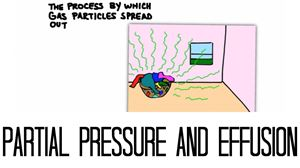This is a two part lesson called Partial Pressure and Effusion.

The first part is called partial pressure. We will learn what partial pressure is and learn how to use three different equations that describe partial pressure.

The second part is called effusion. We will learn how gases effuse and diffuse and then learn how to compare the rate of effusion between different gases.

So good luck and have fun learning about partial pressure and effusion!

#### 41 Lesson 20 - Exercise Review 11:02

In this video we will review the worksheet for Lesson 20.

#### 42 Lesson 21 - The Properties of water and the Dissolving Process 13:05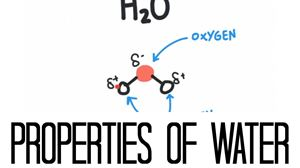This is a two part lesson called Properties of Water and the dissolving process

The first part is called the Properties of Water, we will learn about the properties that make water such a special substance.

The second part is called concentration and solutions where we will learn how a solution is created as well as the different parts of a solution.

So good luck and have fun learning about the properties of water and the dissolving process!

#### 43 Lesson 21 - Exercise Review 08:37

In this video we review the worksheet for Lesson 21.

#### 44 Lesson 22 - Henry's Law and Factors that Affect Solubility 07:46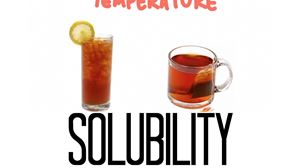This is a two part lesson called Henry’s Law and Factors that Affect Solubility.

The first part is called factors that affect solubility. Where will learn about the factors that affect the solubility of solids and gases in water.

The second part is called Henry’s law where will learn how the pressure of a solution affects the solubility of a gas.

So good luck and have fun learning about Henry’s law and factors that affect solubility!

#### 45 Lesson 22 - Exercise Review 10:49

In this video we review the worksheet for Lesson 22.

#### 46 Lesson 23 - Calculating Concentration 12:36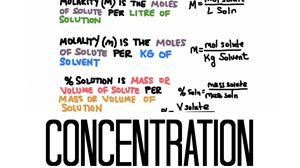This is a one part lesson called Calculating Concentration, where we will learn three different ways to express the concentration of a solution.

So good luck and have fun learning about calculating concentration!

#### 47 Lesson 23 - Exercise Review 12:10

In this video we review the worksheet for Lesson 23.

#### 48 Lesson 24 - Dilutions 06:13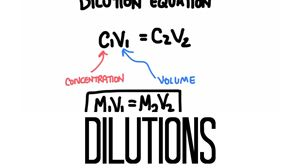This is a one part lesson called Dilutions, where will learn how to calculate and make a dilution from a more concentrated solution.

So good luck and have fun learning about dilutions!

#### 49 Lesson 24 - Exercise Review 10:16

In this video we review the worksheet for Lesson 24.

#### 50 Lesson 25 - Colligative Properties 23:15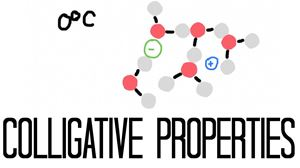This is a two part lesson called colligative properties.

The first part is called colligative properties. We will learn about freezing point depression and boiling point elevation and how to actually calculate the change in freezing point of a solution.

The second part is called determining the molar mass of a solute. We will learn how to determine the molar mass of the solute based on the freezing point depression.

So good luck and have fun learning about colligative properties!

#### 51 Lesson 25 - Exercise Review 12:24

In this video we review the worksheet for Lesson 25.

Course Description

This course covers course content that would appear in the majority of chemistry courses in North America. The course will explore 5 interesting units that are broken up into 25 content-rich lessons. Each lesson includes exercises in the form of worksheets to help students master concepts and practices. In addition to these worksheets, students will find answer videos that will guide students through each question on the worksheets.

Course Goals

Upon completion of this course, students will have the necessary knowledge and skills to advance to grade 12 chemistry. This course is part of a chemistry prerequisite course that many college and university programs require.

Target Audience

This course is for high school students who have already completed an introductory science course that included physical science and life science. Student should have also completed geometry and algebra courses.

Course Requirements

Students should have a good understanding of general science. They should also have completed geometry and algebra courses.

Course Includes

• Over 10 hours of video content

• 25 video lessons

• 25 worksheet review videos

• 25 practice worksheets

• 25 short quizzes

Course Topics

• Properties of matter

• Classification of matter

• Types of mixtures

• Separating mixtures

• Calculating density

• Subatomic particles

• Atomic number, mass number and atomic weight

• Isotopes

• Calculating atomic weight

• Ions

• The periodic table

• Chemical families

• Metals, nonmetals and metalloids

• Periodic trends

• Electronegativity

• Ionization energy

• Ionic compounds and bonding

• Ionic compound nomenclature

• Polyatomic ions

• Covalent compounds and bonding

• Determining a structural formula

• Covalent compound nomenclature

• Molecular shape and polarity

• Intermolecular forces

• Balancing chemical equations

• Synthesis reactions

• Decomposition reactions

• Single and double displacement reactions

• Combustion reactions

• Moles and molar mass of compounds

• Converting the mole

• Calculating percent composition

• Determining an empirical formula

• Determining a molecular formula

• Stoichiometry

• Determining a limiting reactant

• Calculating percent yield

• Kinetic theory

• Solids, liquids and gases

• Converting units of temperature

• Properties of gases

• Heat curves and phase diagrams

• Boyle’s law

• Charles's law

• Gay-Lussac’s law

• Combined gas law

• Ideal gas law

• Dalton’s law of partial pressure

• Graham’s law of effusion

• Properties of water

• The dissolving process

• Solutions

• Factors that affect solubility

• Henry’s law

• Molarity, molality and percent solution

• Dilutions and the dilution equation

• Colligative properties

• Calculating the boiling point elevation of a solution

• Calculating the freezing point depression of a solution

• Teacher: Josh
• Areas of expertise: Chemistry, Biology and Physics.
• Education: B.A. in Chemistry and a B.Ed.
• Interests: Nutrition and fitness. Cross Country Mountain Bike Racing.
• Skills: Chemistry AP Chemistry Physics Technology
• Associations: Ontario College of Teachers. Michigan Certified Teacher. Michigan Science Teacher Association Member.
• Issues I care about: Quality, accessible education for all.

I’m Josh Kenney, I teach high school science and I create online education tutorials. My tutorials are rich with content and yet very accessible to any type of learner. I am a certified teacher in the Province of Ontario and the State of Michigan. In both regions I am certified to teach Chemistry and Biology courses. I have been teaching high school science courses for the past 7 years. I have taught Chemistry, Advanced Placement Chemistry, Health Science, Earth Science, and Physics. I love teaching. I love the process of learning and mastering a subject and I love to participate in that process with my students. I look forward to helping you learn and grow in your knowledge of science!

#### Course Introduction

Course Introduction

#### Answer Key for Lesson Quizzes